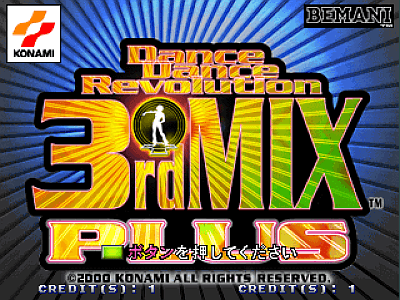# Dance Dance Revolution 3rdMix Plus [Model GCA22]

## Konami System 573 disc+cart. published 20 years ago by Konami## Listed and emulated in MAME !

### Dance Dance Revolution 3rdMix Plus © 2000 Konami Company, Limited.

TECHNICAL
Game ID: GCA22

Runs on the Konami Bemani System 573 Digital Hardware
TRIVIA
Released in June 2000.
SCORING
Grades : SSS - SS - S - A - B - C - D - E
Every song has a maximum of score of 10,000,000 which can only be attained by getting all Perfects. The point value for any step in a combo depends on three factors :
* The length of your combo does not matter.
* Perfects are worth twice as much as Greats.
* The last step in every song is a little bit more important in terms of score. Try to get a Perfect on the last step.

The exact formula for the score of a combo is...
Let N be the number of steps in the song you are playing. Let [x] be the greatest integer function (i.e., [x] = the greatest integer less than or equal to x). Then define b, the point value on which the point value for every step in the song is based, to be :
b = [1,000,000/(N(N+1)/2)]

Then, the point value for the ith step in a combo is :
S(i) = P(i), if the ith step is a Perfect; G(i) if the ith step is a Great;
P(i) = 10bi;
G(i) = 5bi;

The only step that deviates from this formula is the last step, or Nth step, of the song. If you get a Perfect on the Nth step, you get the appropriate points for that Perfect, plus a bonus B :
B(N) = 10,000,000 - (summation of P(i) as i goes from 1 to N)
SERIES
SOURCES
Game's ROM.
Game's screenshots.